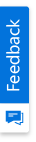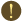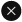We use cookies to give you the best experience on our website. If you continue to browse, then you agree to our privacy policy and cookie policy.Unfortunately, activation email could not send to your email. Please try again.# ASP.NET MVC Combination Chart - Visualize and Compare Data Sets

## Overview

ASP.NET MVC Combination Chart combines two or more chart types into a single chart to compare different data sets. Supports zooming, panning, tooltip, trackball, and selection.

## Combination of line, area, and column chart

You can create multiple series of different types in the same chart. Legend and tooltip for the series make it more readable.

## Pareto chart

Pareto chart is the combination of line and column charts. The column represents data in the descending order, whereas the line chart represents the cumulative value of the data.

## Combination of Candle with Column/Line

You can combine Candle with line or column chart to show additional parameters in financial analysis. For example, volume of the stock can be viewed with the help of column chart.

## ASP.NET MVC Combination Chart Code Example

Easily get started with ASP.NET MVC Combination Chart using a few simple lines of C# code example as demonstrated below. Also explore our ASP.NET MVC Combination Chart Example that shows how to render and configure the chart.

```@Html.EJS().Chart("container").Series(series =>
{
}).PrimaryXAxis(px => px.ValueType(Syncfusion.EJ2.Charts.ValueType.Category)).Render();```
```public class HomeController : Controller
{
public ActionResult Index()
{
List<ChartData> chartData = new List<ChartData>
{
new ChartData{ x=2000, y1= 0.03, y2= 0.48},
new ChartData{ x=2001, y1= 0.05, y2= 0.53 },
new ChartData{ x=2002, y1= 0.06, y2= 0.57 },
new ChartData{ x=2003, y1= 0.09, y2= 0.61 },
new ChartData{ x=2004, y1= 0.14, y2= 0.63 },
new ChartData{ x=2005, y1= 0.20, y2= 0.64 },
new ChartData{ x=2006, y1= 0.29, y2= 0.66 },
new ChartData{ x=2007, y1= 0.46, y2= 0.76 },
new ChartData{ x=2008, y1= 0.64, y2= 0.77 },
new ChartData{ x=2009,  y1= 0.75, y2= 0.55 }
};
ViewBag.dataSource = chartData;
return View();
}
}
public class ChartData
{
public double x;
public double y1;
public double y2;
}```

## Learning ResourcesCombination Chart User Guide

Learn the available options to customize ASP.NET MVC combination chart.Combination Chart API Reference

Explore the ASP.NET MVC combination chart APIs.

### 80+ ASP.NET MVC UI CONTROLS

ALL CONTROLS
VIEWERYou are using an outdated version of Internet Explorer that may not display all features of this and other websites. Upgrade to Internet Explorer 8 or newer for a better experience.